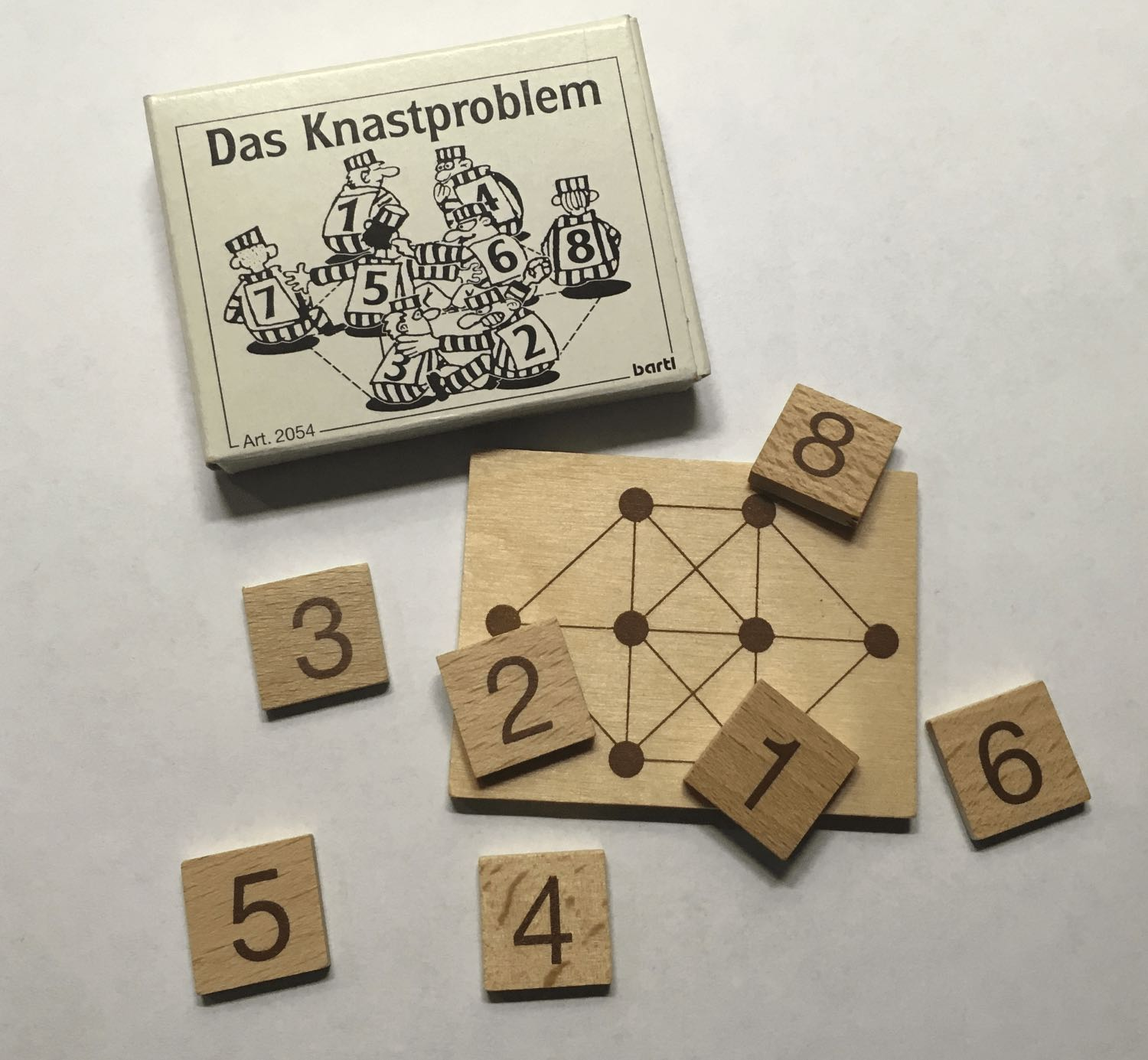# Calculating permutations with C# and LINQ

Recently I got this puzzle game and after solving it for the second time, I could not remember if I used the same solution twice. I was wondering if there is only one solution and decided to spend way too much time writing a solver in C#.Knastproblem is a puzzle game where you have to place eight inmates at eight seats in the mess hall. The rules are that you cannot put inmates with adjacent numbers at adjacent seats. A typical permutation game where you have to re-order a given combination of numbers. From wiki:

In mathematics, the notion of permutation relates to the act of arranging all the members of a set into some sequence or order

My first task was to calculate all the possible seating positions without checking if the rules are violated. For eight inmates that would be 8! The exclamation mark indicates a factorial and 8! translates to 1x2x3x4x5x6x7x8 = 40320.

## Calculating permutations

This stackoverflow article showed me a few solutions and I decided to write my own C# version using LINQ and recursion:

``````static void Main(string[] args)
{
int counter = 1;
foreach (var p in Permutate("ABC"))
{
Console.WriteLine(\$"{counter++}: {p}");
}
}

private static IEnumerable<string> Permutate(string source)
{
if (source.Length == 1) return new List<string> { source };

var permutations = from c in source
from p in Permutate(new String(source.Where(x => x != c).ToArray()))
select c + p;

return permutations;
}

``````

Running this code resulted in:Calculating six permutations using `"ABC"` as input was fast. Using `"ABCDEFGH"` with 40320 permutations took a while so if speed is important to you, this solution is probably not the one you are looking for. But for my case it was sufficient and after a while I found all the combinations:My next step is to apply the rules of the game but that is out of the scope of this post. My goal was to share my solution to calculate permutations with C# and LINQ

Grüsse aus Berlin!
This is a cookie free and popup free website.Join me in making the web enjoyable again by removing the need for annoying cookie warnings and silly newsletter overlays!